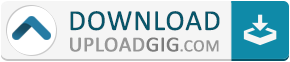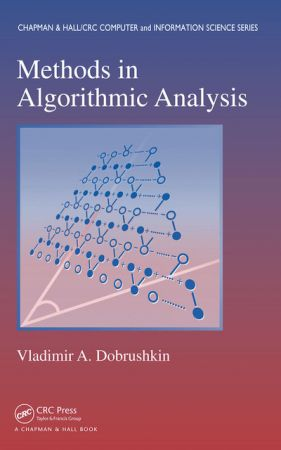#Do not remember me!

### Categories» » » Methods in Algorithmic Analysis

## Methods in Algorithmic AnalysisEnglish | 2016 | ISBN: 9781420068306 | 824 ages | EPUB | 5 MB

Explores the Impact of the Analysis of Algorithms on Many Areas within and beyond Computer Science
A flexible, interactive teaching format enhanced by a large selection of examples and exercises

Developed from the author’s own graduate-level course, Methods in Algorithmic Analysis presents numerous theories, techniques, and methods used for analyzing algorithms. It exposes students to mathematical techniques and methods that are practical and relevant to theoretical aspects of computer science.

After introducing basic mathematical and combinatorial methods, the text focuses on various aspects of probability, including finite sets, random variables, distributions, Bayes’ theorem, and Chebyshev inequality. It explores the role of recurrences in computer science, numerical analysis, engineering, and discrete mathematics applications. The author then describes the powerful tool of generating functions, which is demonstrated in enumeration problems, such as probabilistic algorithms, compositions and partitions of integers, and shuffling. He also discusses the symbolic method, the principle of inclusion and exclusion, and its applications. The book goes on to show how strings can be manipulated and counted, how the finite state machine and Markov chains can help solve probabilistic and combinatorial problems, how to derive asymptotic results, and how convergence and singularities play leading roles in deducing asymptotic information from generating functions. The final chapter presents the definitions and properties of the mathematical infrastructure needed to accommodate generating functions.

Accompanied by more than 1,000 examples and exercises, this comprehensive, classroom-tested text develops students’ understanding of the mathematical methodology behind the analysis of algorithms. It emphasizes the important relation between continuous (classical) mathematics and discrete mathematics, which is the basis of computer science.Name:* E-Mail: Security Code: *